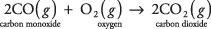## Chemical Reactions

The standard representation of a chemical reaction shows an arrow pointing from the reactants to the products:For most chemical reactions in this book, solids are labeled (s), liquids (l), and gases (g). The numerical coefficients in front of the chemical formulas express the moles of each compound or element. The preceding reaction can be interpreted in terms of moles or masses. (See Table 1.)Notice that the total mass of the reactants (243.73 g) equals the total mass of the products. This demonstrates the law of conservation of mass, which applies to all chemical reactions.

It is not true, however, that volumes must be conserved in reactions involving gases. The complete combustion of carbon monoxide is a case in point:Because the reaction coefficients are proportional to relative volumes of each gas, 2 volumes of carbon monoxide and 1 volume of oxygen (a total of 3 volumes of reactants) combine to produce only 2 volumes of carbon dioxide. In gaseous reactions, the total volume of the products may be less than, or equal to, or greater than the total volume of the reactants.

• How many liters of oxygen (O 2) are required for the complete oxidation of 1 gram of methane when the following reaction occurs at STP?